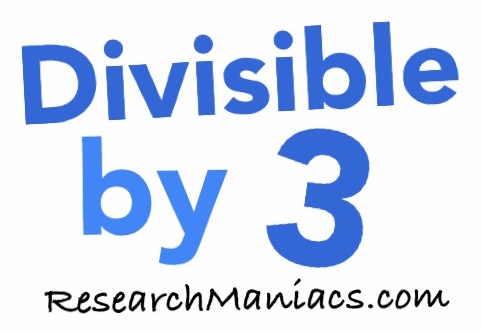Is 19 divisible by 3?Is 19 divisible by 3? In other words, if you divide 19 by 3, will you get a whole number with no remainder?

Of course, you could use a calculator to find out if 19 is divisible by 3, but what fun would that be?

To find out if 19 is divisible by 3, we will add up the numbers that make 19 as follows: 1 + 9 = 10

We know that if the sum of the numbers that make up 19 is divisible by 3, then 19 is divisible by 3.

Since the sum of the digits in 19 is not divisible by 3, 19 is also NOT divisible by 3. Thus, the answer to the question "Is 19 divisible by 3?" is as follows:

No

Note: If you divide 19 by 3, you get 6.33 which is not a whole number.

Divisible by 3?
Do you need to check another number? Enter a number below to see if it is divisible by 3.

Is divisible by 3?

Is 20 divisible by 3?
Can you do it on your own now? If not, go here for the next number on our list.